# Coulomb's Law

## Like charges repel, unlike charges attract.

The electric force acting on a point charge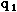as a result of the presence of a second point charge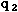is given by Coulomb's Law: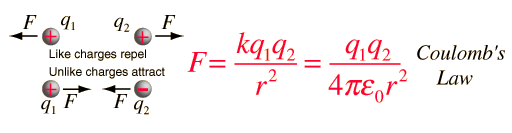where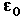permittivity of space

Note that this satisfies Newton's third law because it implies that exactly the same magnitude of force acts on. Coulomb's law is a vector equation and includes the fact that the force acts along the line joining the charges. Like charges repel and unlike charges attract. Coulomb's law describes a force of infinite range which obeys the inverse square law, and is of the same form as the gravity force.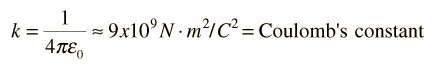Forx10^ Cx 10^ Cand r meters,F x 10^ Newtons. A negative force implies an attractive force. The force is directed along the line joining the two charges.
Index

Electromagnetic force
HyperPhysics***** Electricity and Magnetism Go Back

# Electric Force Example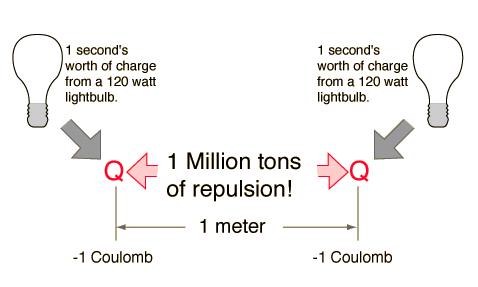The electric force between charges may be calculated using Coulomb's law.

Index

Electromagnetic force
HyperPhysics***** Electricity and Magnetism Go Back

# Coulomb's ConstantThe constant of proportionality appearing in Coulomb's law is often called Coulomb's constant. Note that it can be expressed in terms of another constant,permittivity of space.

When describing the electric forces in atoms and nuclei, it is often convenient to work with the product of Coulomb's constant and the square of the electron charge since that product appears in electric potential energy and electric force expressions. That product in units appropriate for atomic and nuclear processes is: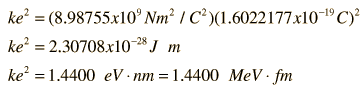The electric force between charges may be calculated using Coulomb's law.

Index

Electromagnetic force
HyperPhysics***** Electricity and Magnetism Go Back